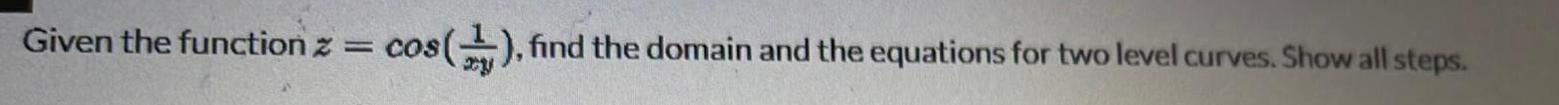Question:

# Given the function z cos find the domain and the equations

Last updated: 5/11/2023Given the function z cos find the domain and the equations for two level curves Show all steps